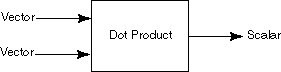created 08/26/97; revised 08/12/00, 07/23/0, 07/23/09, 01/11/15

# CHAPTER 7 — Dot Product

Examine the operations that have been discussed so far:Vector addition: vector + vector = vector Vector subtraction: vector - vector = vector Difference of points: point - point = vector Scaling: real * vector = vector

This chapter discusses the dot product, which takes two vectors as operands and produces a real number as its output. Sometimes the dot product is called the inner product. Sometimes the dot product is called the scalar product, which should not be confused with the operation called scaling (but probably will be).

 Dot Product: vector · vector = scalar

### QUESTION 1:

Why is the dot product sometimes called the scalar product?